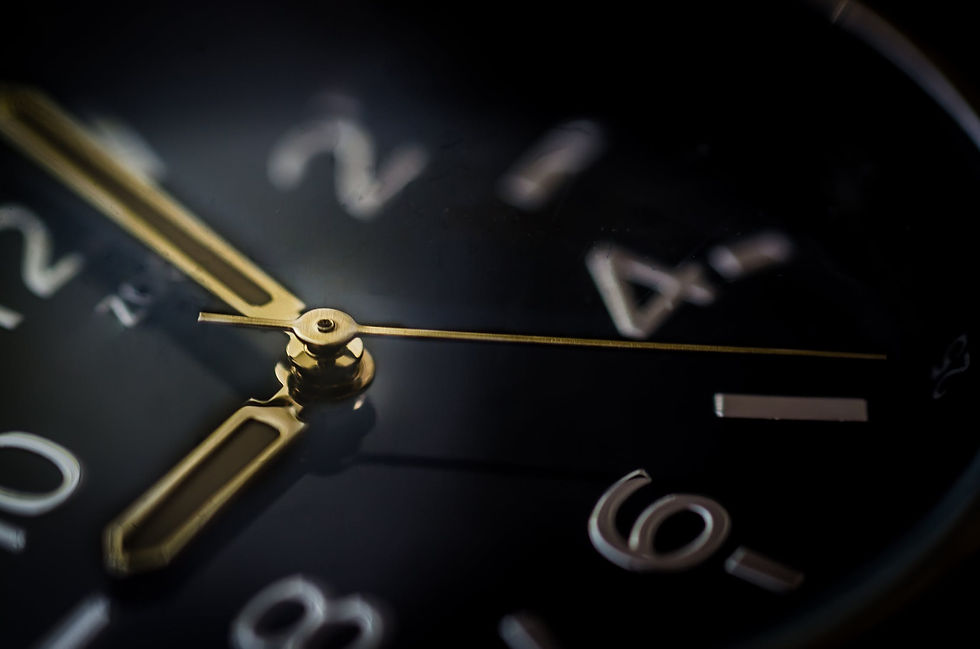top of page
Search

# What is the angle between the hour and the minutes hand when the time is 4.40 pm?

Updated: Sep 17, 2018

Overview of City Investment TrainingWe have a 89% placement success rate for students on our 8 week training program. Click on the link below to find out how our training and internship programs can help you launch a career in investment banking.

What is the angle between the hour and the minutes hand when the time is 4.40pm?

To tee off, what will be handy is to first compute the angles for every one hour or five minutes. From there on we can work out the angles for any time.

The number of degrees in one hour

We know that a circle consists of 360 degrees.

Thee clock has 12 hours, so we need to divide 360 by 12 to get the degree for every hour or five minutes.

i.e. 360/12 we get 30 degrees in every one hour or five minutes

The angle between 4 hour and 40 minutes

We know that the minutes hand at 40 minutes means the same as the hour hand at 8 pm.

So, for working out the angle for 4:40 we get

8 – 4 =4 hours

The angle for four hours is 4*30 degrees = 120 degrees

Be careful – it ain’t over – this is what separates a good candidate from a great one.

The angle at 4.40pm

Remember at time 4:40, the hour hand does not stand still at 4 but has started to make its way toward 5. The question is how much?

If the time is 4:40 then the minutes hand has moved 2/3rd its way towards 12 and so the hours hand would have moved by the same proportion i.e. 2/3rd which gives us an angle of 2/3rd of an hour or 2/3rd of 30 degrees i.e. 20 degrees.

We will now subtract this from 120 degrees to get 100 degrees.